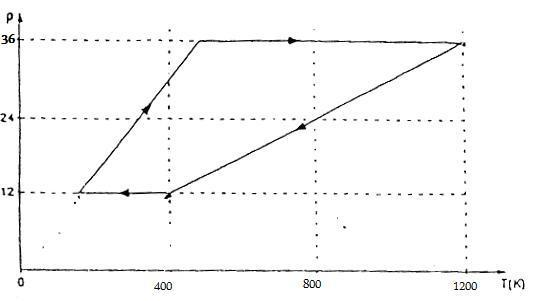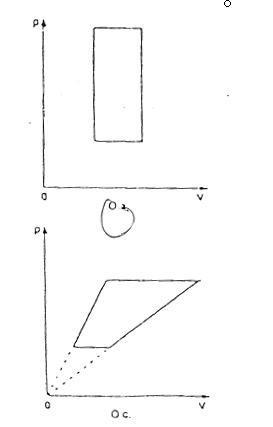# Ideal gas undergoes cycle process

Hi,

An amount of ideal gas undergoes the folowing cycle process in the following graph:This process can be presented by the following PV-graph :The answer has to be the upper graph but why can't it be the lower graph ?
Thank you!

#### Attachments

I like Serena
Homework Helper
An ideal gas has: p V = n R T

That is: V = n R T / p

As you can see in the upper graph there are linear relations between T and p, meaning V must be constant, resulting in vertical lines in the p-V-graph.
Note that the diagonal lines in the upper graph can be extended to intersect the axes at the origin.